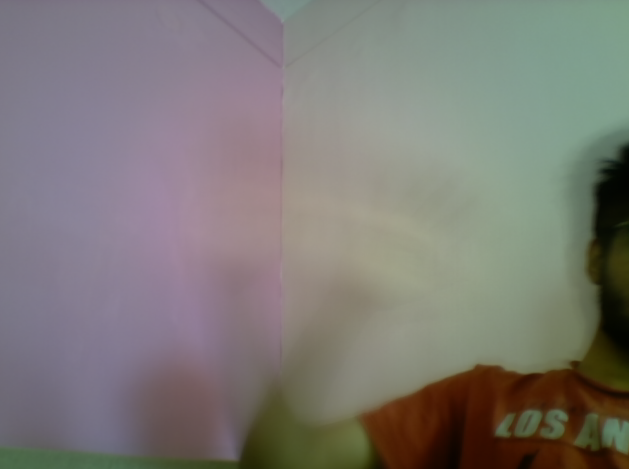GFG App
Open AppBrowser
Continue

# Background Subtraction in an Image using Concept of Running Average

Background subtraction is a technique for separating out foreground elements from the background and is done by generating a foreground mask. This technique is used for detecting dynamically moving objects from static cameras. Background subtraction technique is important for object tracking. There are several techniques for background subtraction

In this article, we discuss the concept of Running Average. The running average of a function is used to separate foreground from background. In this concept, the video sequence is analyzed over a particular set of frames. During this sequence of frames, the running average over the current frame and the previous frames is computed. This gives us the background model and any new object introduced in the during the sequencing of the video becomes the part of the foreground. Then, the current frame holds the newly introduced object with the background. Then the computation of the absolute difference between the background model (which is a function of time) and the current frame (which is newly introduced object) is done. Running average is computed using the equation given below :

``````

Prerequisites :

• A working web camera or a camera module for input.
• Download Python 3.x, Numpy and OpenCV 2.7.x version. Check if your OS is either 32 bit or 64 bit compatible and install accordingly.
• Check the running status of numpy and OpenCV

How Running Average method works?

The objective of the program is to detect active objects from the difference obtained from the reference frame and the current frame. We keep feeding each frame to the given function, and the function keeps finding the averages of all frames. Then we compute the absolute difference between the frames.
The function used is cv2.accumulateWeighted().

```
cv2.accumulateWeighted(src, dst, alpha)

```

The parameters passed in this function are :

1. src: The source image. The image can be colored or grayscaled image and either 8-bit or 32-bit floating point.
2. dst: The accumulator or the destination image. It is either 32-bit or 64-bit floating point.
NOTE: It should have the same channels as that of the source image. Also, the value of dst should be predeclared initially.
3. alpha: Weight of the input image. Alpha decides the speed of updating. If you set a lower value for this variable, running average will be performed over a larger amount of previous frames and vice-versa.

Code:

 `# Python program to illustrate ` `# Background subtraction using ` `# concept of Running Averages ` ` `  `# organize imports ` `import` `cv2 ` `import` `numpy as np ` ` `  `# capture frames from a camera ` `cap ``=` `cv2.VideoCapture(``0``) ` ` `  `# read the frames from the camera ` `_, img ``=` `cap.read() ` ` `  `# modify the data type ` `# setting to 32-bit floating point ` `averageValue1 ``=` `np.float32(img) ` ` `  `# loop runs if capturing has been initialized.  ` `while``(``1``): ` `    ``# reads frames from a camera  ` `    ``_, img ``=` `cap.read() ` `     `  `    ``# using the cv2.accumulateWeighted() function ` `    ``# that updates the running average ` `    ``cv2.accumulateWeighted(img, averageValue1, ``0.02``) ` `     `  `    ``# converting the matrix elements to absolute values  ` `    ``# and converting the result to 8-bit.  ` `    ``resultingFrames1 ``=` `cv2.convertScaleAbs(averageValue1) ` ` `  `    ``# Show two output windows ` `    ``# the input / original frames window ` `    ``cv2.imshow(``'InputWindow'``, img) ` ` `  `    ``# the window showing output of alpha value 0.02 ` `    ``cv2.imshow(``'averageValue1'``, resultingFrames1) ` `     `  `    ``# Wait for Esc key to stop the program  ` `    ``k ``=` `cv2.waitKey(``30``) & ``0xff` `    ``if` `k ``=``=` `27``:  ` `        ``break` ` `  `# Close the window  ` `cap.release()  ` `   `  `# De-allocate any associated memory usage  ` `cv2.destroyAllWindows() `

Output :

As we can see clearly below, the hand blocks the background view.Now, we shake the foreground object i.e. our hand. We begin waving our hand.

The Running average shows the background clearly below, Running Average with alpha 0.02 has caught it as a transparent hand, with main emphasis on the backgroundAlternatively, we can use the cv.RunningAvg() for the same task with the parameters having the same meaning as that of the parameters of the cv2.accumulateweighted().

```
cv.RunningAvg(image, acc, alpha)

```

References :

1. https://docs.opencv.org/2.4/modules/imgproc/doc/motion_analysis_and_object_tracking.html
2. https://en.wikipedia.org/wiki/Foreground_detection
3. https://docs.opencv.org/3.2.0/d1/dc5/tutorial_background_subtraction.html

My Personal Notes arrow_drop_up